# AQA A Level Physics复习笔记3.4.2 The Diffraction Grating

### The Diffraction Grating

• A diffraction grating is a plate on which there is a very large number of parallel, identical, close-spaced slits
• When monochromatic light is incident on a grating, a pattern of narrow bright fringes is produced on a screenDiagram of diffraction grating used to obtain a fringe pattern

• The angles at which the maxima of intensity (constructive interference) are produced can be deduced by the diffraction grating equation:• Exam questions sometime state the lines per m (or per mm, per nm etc.) on the grating which is represented by the symbol N
• d can be calculated from N using the equation#### Angular Separation

• The angular separation of each maxima is calculated by rearranging the grating equation to make θ the subject
• The angle θ is taken from the centre meaning the higher orders are at greater angles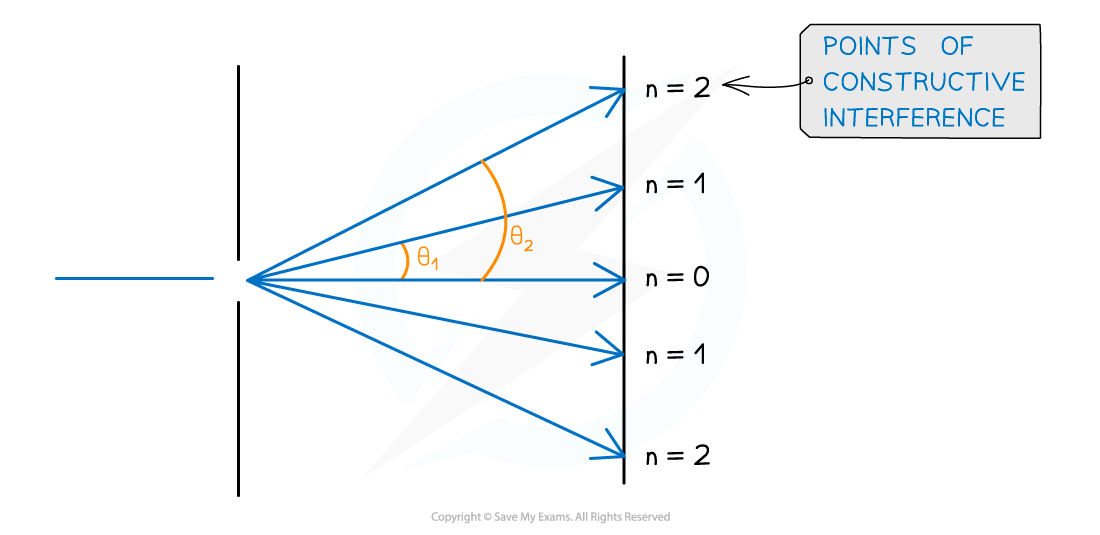Angular separation

• The angular separation between two angles is found by subtracting the smaller angle from the larger one
• The angular separation between the first and second maxima n1 and n2 is θ2 – θ1

#### Orders of Maxima

• The maximum angle to see orders of maxima is when the beam is at right angles to the diffraction grating
• This means θ = 90o and sin θ = 1

• The highest order of maxima visible is therefore calculated by the equation:• Note that since n must be an integer, if the value is a decimal it must be rounded down
• E.g If n is calculated as 2.7 then n = 2 is the highest order visible

#### Worked Example

An experiment was set up to investigate light passing through a diffraction grating with a slit spacing of 1.7 µm. The fringe pattern was observed on a screen. The wavelength of the light is 550 nm.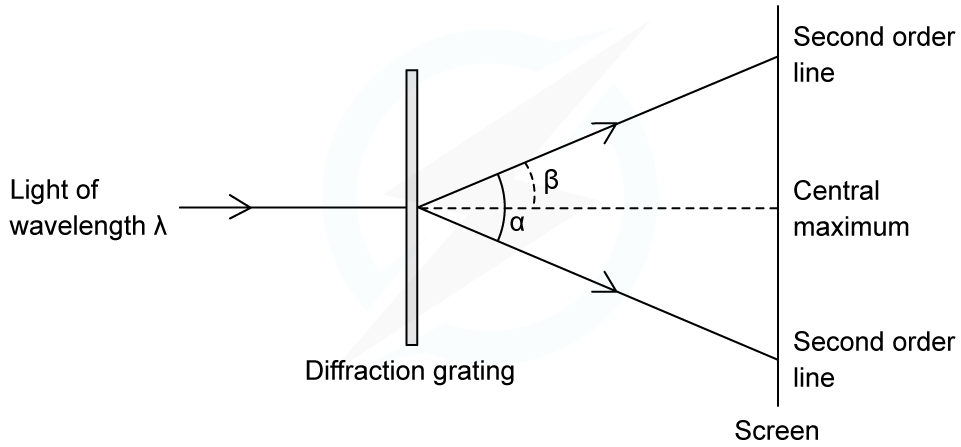Calculate the angle α between the two second-order lines.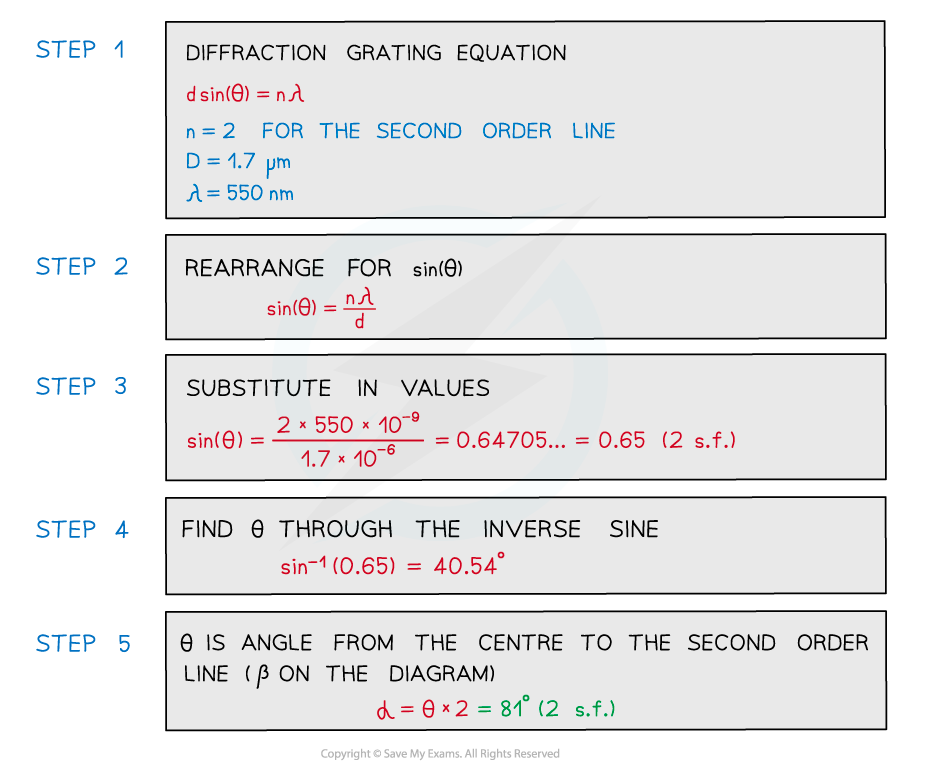#### Exam Tip

Take care that the angle θ is the correct angle taken from the centre and not the angle taken between two orders of maxima.

### Derivation of the Diffraction Grating Equation

• When light passes through the slits of the diffraction grating, the path difference at the zeroth order maximum is zero
• At the first order maxima, there is constructive interference, hence the path difference is λ
• Therefore, at the nth order maxima, the path difference is equal to nλ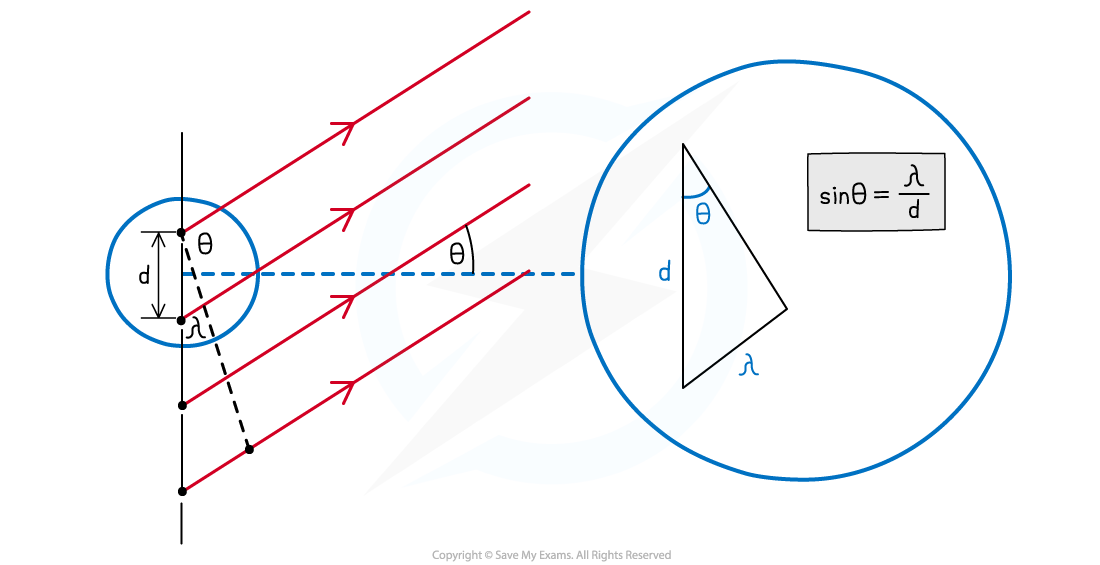Using this diagram and trigonometry, the diffraction grating equation can be derived

• Using trigonometry, an expression for the first order maxima can be written:• Where:
• θ = the angle between the normal and the maxima
• λ = the wavelength of the light (m)
• d = the slit separation (m)

• This means, for n = 1:• Similarly, for n = 2, where the path difference is 2λ:• Therefore, in general, where the path difference is nλ:• A small rearrangement leads to the equation for the diffraction grating:

### Applications of Diffraction Gratings

• Diffraction gratings are useful for separating light of different wavelengths with high resolution
• They are used in spectrometers to:
• Analyse light from stars
• Analyse the composition of a star
• Chemical analysis
• Measure red shift / rotation of stars
• Measure the wavelength / frequency of light from a star
• Observe the spectra of materials
• Analyse the absorption / emission spectra in stars

• Diffraction gratings also play a role in x-ray crystallography
• X-rays are directed at a thin crystal sheet which acts as a diffraction grating to form a diffraction pattern
• This is because the wavelength of x-rays is similar in size to the gaps between the atoms
• This diffraction pattern can be used to measure the atomic spacing in certain materials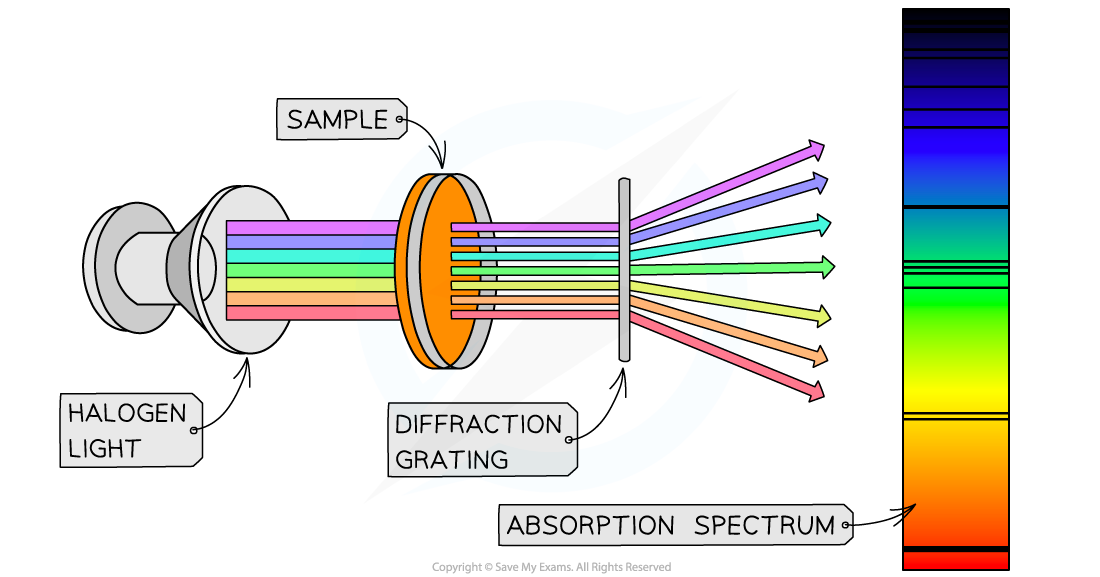Diffraction gratings are most commonly used in spectrometers. These devices play a crucial role in areas of physics such as atomic physics and astrophysics

#### Exam Tip

Note that while you will be expected to know some applications of diffraction gratings, you will not be tested on specific apparatus or techniques such as spectrometry.When describing the use of spectrometers, avoiding using vague terms such as observe spectra’, ‘spectroscopy’, ‘view absorption / emission spectrum’, ‘compare spectra’, ‘look at light from stars’.